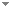Switch to: References

# Some properties of r-maximal sets and Q 1,N -reducibility

Archive for Mathematical Logic 54 (7-8):941-959 (2015)

 $$sQ_1$$-degrees of computably enumerable sets.Roland Sh Omanadze - forthcoming - Archive for Mathematical Logic:1-17.We show that the sQ-degree of a hypersimple set includes an infinite collection of $$sQ_1$$ -degrees linearly ordered under $$\le _{sQ_1}$$ with order type of the integers and each c.e. set in these sQ-degrees is a hypersimple set. Also, we prove that there exist two c.e. sets having no least upper bound on the $$sQ_1$$ -reducibility ordering. We show that the c.e. $$sQ_1$$ -degrees are not dense and if a is a c.e. $$sQ_1$$ -degree such that $$o_{sQ_1}<_{sQ_1}a<_{sQ_1}o'_{sQ_1}$$, then there exist (...)No categories Direct download (2 more)     Export citationBookmark$$sQ_1$$ s Q 1 -degrees of computably enumerable sets.Roland Sh Omanadze - forthcoming - Archive for Mathematical Logic:1-17.We show that the sQ-degree of a hypersimple set includes an infinite collection of \-degrees linearly ordered under \ with order type of the integers and each c.e. set in these sQ-degrees is a hypersimple set. Also, we prove that there exist two c.e. sets having no least upper bound on the \-reducibility ordering. We show that the c.e. \-degrees are not dense and if a is a c.e. \-degree such that \, then there exist infinitely many pairwise sQ-incomputable c.e. (...)No categories Direct download (3 more)     Export citationBookmarkr ‐Maximal sets and Q1,N‐reducibility.Roland Sh Omanadze & Irakli O. Chitaia - forthcoming - Mathematical Logic Quarterly.No categories Direct download     Export citationBookmark